Next: 7.2 Five-Stage CMOS Ring Up: 7.1 Devices Previous: 7.1.1 MOS Transistors

## 7.1.2 Silicon-Germanium HBT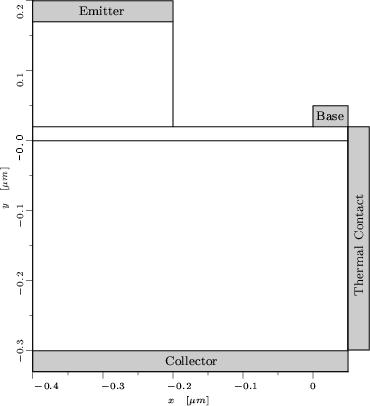As a bipolar device the SiGe heterojunction bipolar transistor (HBT) as shown in Fig. 7.1 was chosen which was published in . It has a constant Ge doping in the base with a Ge mole fraction x of 0.2. The doping profile is shown in Fig. 7.2. The main advantage of this device design is the high emitter efficiency which can be obtained. This is due to energy gap difference between the emitter and base materials. In traditional homojunction transistors, the ratio of the doping concentrations in the emitter and base must be very high. This layout allows for a very high base doping to reduce the base resistance and a very thin base to reduce base transit time. Furthermore, the emitter may be lightly doped to reduce the base-emitter junction capacity without degrading performance. The current gain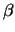can easily be adjusted by modifying the Ge mole fraction x. This has an important impact on the numerical behavior of the circuits as the current gain determines the coupling of the single stages of the circuits.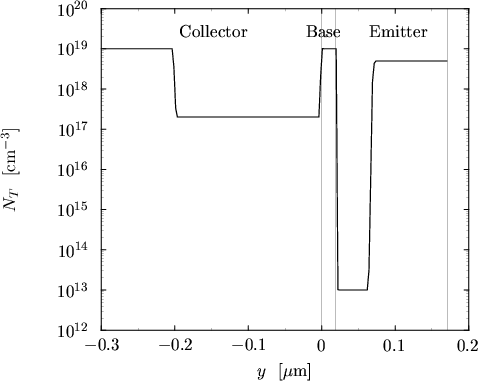The mobility model used is very similar to the model discussed in Chapter 3. However, to account for different Ge contents, it makes use of two different parameter sets, one for Si and one for Ge to calculate the two low-field mobilities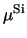and. These low-field mobilities are combined by a harmonic mean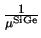=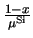++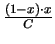(7.1)

with C being the bowing parameter.

For the high-field mobility model the saturation velocities of the basic materials are interpolated quadratically

 v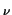SiGe = vSi . (1 - x) + vGe . x + C . (1 - x) . x (7.2)

The most important effect when comparing DD to HD simulations is the velocity overshoot occurring in the base-collector space charge region. This is due to a maximum of the electric field which occurs in the base-collector junction for normal forward operation of the transistor as shown in Fig. 7.3. No difference in the electric field was observed between both transport models. However, the velocity shown in Fig. 7.4 for different base-collector voltages VBC and VBE = 0.75 V shows a significant overshoot for the HD model. The velocities obtained with the DD model vn are strictly limited by the saturation velocity vsat = 107 cm/s. The overshoot occurs both in space and in time, resulting in an increased speed of the devices. A plot of the simulated electron temperature is shown in Fig. 7.5. The maximum of the temperature is shifted into the collector region as the carrier temperature cannot follow the high-field immediately. Velocity overshoot occurs in this region where the electric field is high and the temperature is only beginning to rise.

For holes similar velocity and temperature distributions were obtained. However, as the hole concentration in the collector is extremely low this had no impact on the solution. Hence, in all HD mixed-mode simulations the hole temperature Tp was set to TL which is equivalent to performing a DD simulation without considering velocity saturation in the mobility model. This approach has two benefits. First, the matrix size is reduced by 1/5 and secondly because calculating Tp deteriorates the convergence rate of the whole system. Convergence could only be obtained by applying a three step iteration scheme: in the first block the DD equations were solved, followed by the HD equations without considering the hole temperature in the second block and only in the final block it was possible to add the hole temperature to the equation system. This final block took as many iterations as the first two blocks and only marginally changed the solution well below the accuracy of the linear solver.

The following simulations served to judge the influence of the thermal contact conductances when performing mixed-mode self-heating simulations. As a thermal contact an isothermal silicon block was added at the right side of the device. In Fig. 7.6 the temperature of this thermal contact is shown in dependence of the thermal contact conductance Gth. For a moderate VCE = 2 V the contact temperature is very sensitive to the contact conductance while the collector and base current change only marginally as shown in Fig. 7.7. Hence, the current gain does not change noticeably for different values of Gth as shown in Fig. 7.7. This must not be confused with the strong contact temperature dependence of the current gain as shown in Fig. 7.9. This can occur when the contact temperature is determined by another device. This issue is of importance when simulating thermal coupling of devices.

The final simulation aimed to determine the self-heating time constant of the HBT. This was done by calculating the operating point without self-heating and turning self-heating on for the transient analysis which gave the fictitious step response of the lattice heat flow equation. The time constant was found to be approximately 1.8 ns. This time constant can be approximated using the thermal resistance and capacity of the material. With the expressions from Appendix A

 Rth =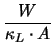(7.3) Cth = A . W .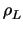. cL (7.4)

and the values from the MINIMOS-NT material database with W = 0.5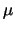m and A = 0.4m2 one obtains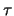= Rth . Cth = 4.3 ns (7.5)

which is of the same order as the simulated value.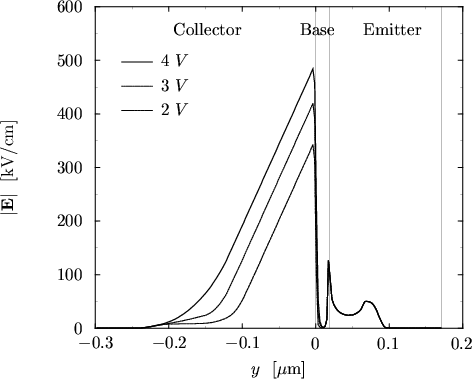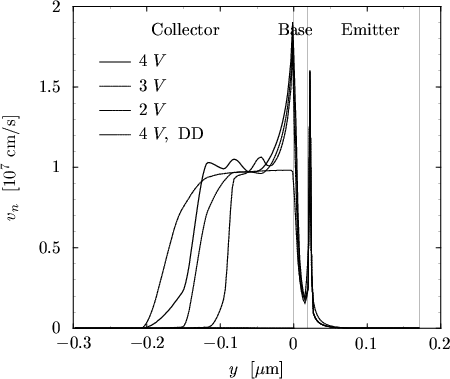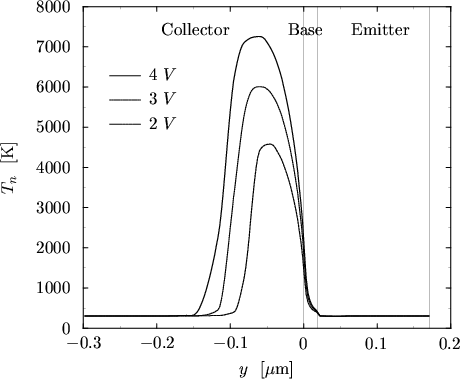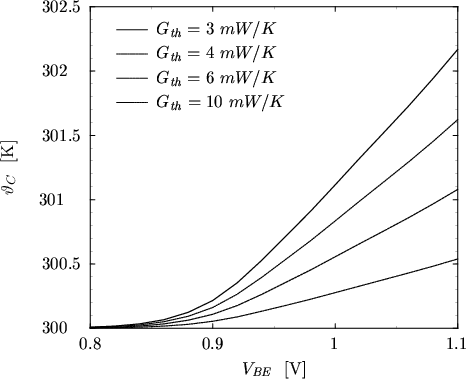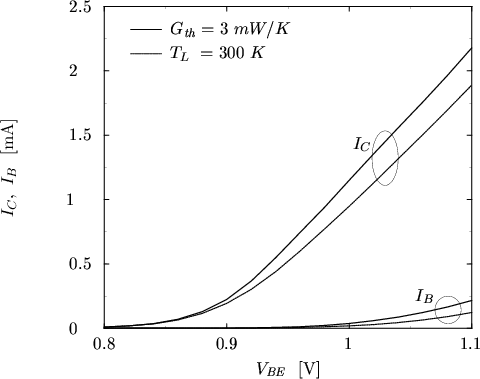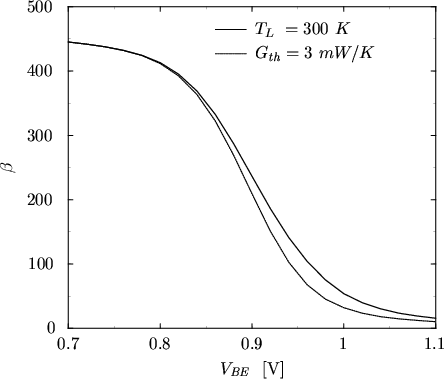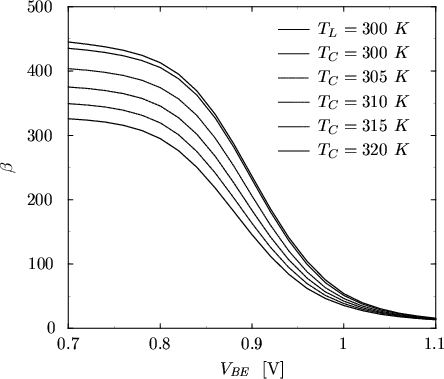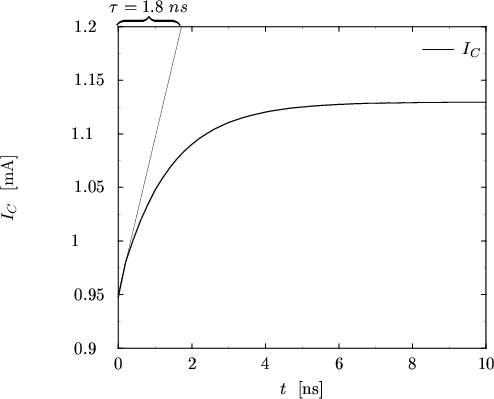Next: 7.2 Five-Stage CMOS Ring Up: 7.1 Devices Previous: 7.1.1 MOS Transistors
Tibor Grasser
1999-05-31